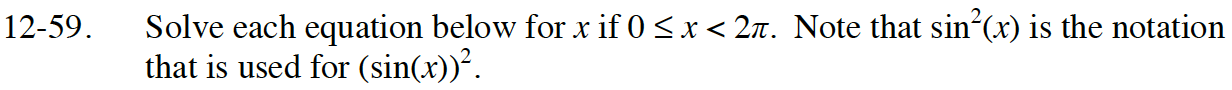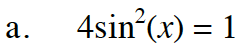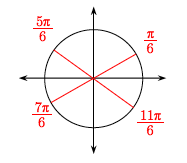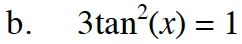### Home > INT3 > Chapter 12 > Lesson 12.1.4 > Problem12-59

12-59.
1. Solve each equation below for x if 0 ≤ x < 2π. Note that sin2(x) is the notation that is used for (sin(x))2. Homework Help ✎

1. 4sin2(x) = 1

2. 3tan2(x) = 1Divide both sides by 4.

$\sin^2(x)=\frac{1}{4}$

Take the square root of both sides to solve for sin(x).

$\sin(x)=±\frac{1}{2}$

Find the corresponding angles on the unit circle.Follow the steps in part (a).